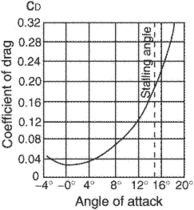# coefficient of drag

Also found in: Dictionary, Thesaurus, Acronyms, Wikipedia.
Related to coefficient of drag: Coefficient of lift

## coefficient of drag (CD)A coefficient dependent upon the angle of attack, shape of the airfoil, Reynolds number, and Mach number. Drag coefficient (CD) = Drag (D)/Dynamic Pressure (q) or {¼(Density (ρ) × Air Speed2 (V2)} x Wing Area (S). CD = D/qS, or 2D/ρV2S.
References in periodicals archive ?
The specific inter-vehicle distance of "long" will be described in detail later), the coefficient of drag [C.sub.D1] and projected area [A.sub.f1] of the leading vehicle can be substituted into Equations (1) through (3) as before to determine the aerodynamic drag reduction rate of the trailing vehicle even when the leading vehicle is of a different type.
The aerodynamic design of the 2010 Tahoe results in a segment-leading coefficient of drag of 0.
With a coefficient of drag of 0.26, it is better than sleek sports cars like the Porsche 911 and Chevrolet Corvette.
And when it was tested in a wind tunnel the company's hunch was confirmed, with the lowest possible coefficient of drag, or air resistance, for a vehicle of its size.
Here [rho] is the density of seawater (nominally 1025 kg [m.sup.-3]), U is water velocity (m [s.sup.-1]), S is maximal planform area ([m.sup.2], essentially half the wetted surface area of the frond), and [C.sub.d] is the dimensionless coefficient of drag. In a comparison of groups of algae taken from "exposed" and "protected" sites, Carrington (1990) found no significant difference in stipe strength (breaking force divided by cross-sectional area at the breaking point) for M.
To calculate the coefficient of drag, the Wrights created a "drift balance" to measure an angle they called the tangential, which "gives the inclination of the chord [of the wing] above or below the horizon."
The new Accord also features an aerodynamic design and resultant high fuel efficiency with a coefficient of drag value of 0.26 -- among the world's highest ratings for a sedan.
With less frontal area and a slightly smaller coefficient of drag, the Dakota requires less power to reach 210 mph than the larger Dodge Ram package.
This low coefficient of drag (LCD) patented technology is said to produce a durable, flexible and lightweight hose unparalleled in the material handling industry, according to the literature.
The coefficient [Beta.sub.d] is the velocity exponent of drag and [S.sub.d,pr] is the shape coefficient of drag, defined using the profile area, [A.sub.pr], the organism's area projected onto a plane perpendicular to the direction of water motion.

Site: Follow: Share:
Open / Close